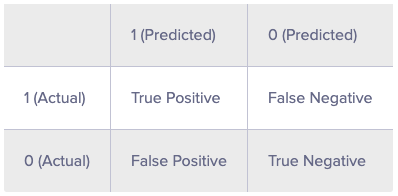# Create a Confusion Matrix in R

A confusion matrix is a tabular representation of Actual vs Predicted values.As you can see, the confusion matrix avoids "confusion" by measuring the actual and predicted values in a tabular format. In table above, Positive class = 1 and Negative class = 0. Following are the metrics we can derive from a confusion matrix:

Accuracy - It determines the overall predicted accuracy of the model. It is calculated as Accuracy = (True Positives + True Negatives)/(True Positives + True Negatives + False Positives + False Negatives)

True Positive Rate (TPR) - It indicates how many positive values, out of all the positive values, have been correctly predicted. The formula to calculate the true positive rate is (TP/TP + FN). Also, TPR = 1 - False Negative Rate. It is also known as Sensitivity or Recall.

False Positive Rate (FPR) - It indicates how many negative values, out of all the negative values, have been incorrectly predicted. The formula to calculate the false positive rate is (FP/FP + TN). Also, FPR = 1 - True Negative Rate.

True Negative Rate (TNR) - It indicates how many negative values, out of all the negative values, have been correctly predicted. The formula to calculate the true negative rate is (TN/TN + FP). It is also known as Specificity.

False Negative Rate (FNR) - It indicates how many positive values, out of all the positive values, have been incorrectly predicted. The formula to calculate false negative rate is (FN/FN + TP).

Precision: It indicates how many values, out of all the predicted positive values, are actually positive. It is formulated as:(TP / TP + FP).

F Score: F score is the harmonic mean of precision and recall. It lies between 0 and 1. Higher the value, better the model. It is formulated as 2((precision*recall) / (precision+recall)).

We can create the confusion matrix for our data.

> confusionMatrix(credit_test\$Creditability,pred_value_labels)
Confusion Matrix and Statistics
Reference
Prediction   0   1
0  48  32
1  59 161
Accuracy : 0.6967
95% CI : (0.6412, 0.7482)
No Information Rate : 0.6433
P-Value [Acc > NIR] : 0.02975
Kappa : 0.2996
Mcnemar's Test P-Value : 0.00642
Sensitivity : 0.4486
Specificity : 0.8342
Pos Pred Value : 0.6000
Neg Pred Value : 0.7318
Prevalence : 0.3567
Detection Rate : 0.1600
Detection Prevalence : 0.2667
Balanced Accuracy : 0.6414
'Positive' Class : 0